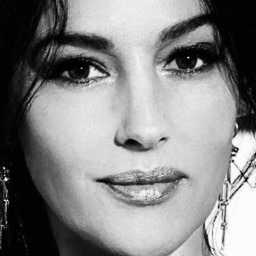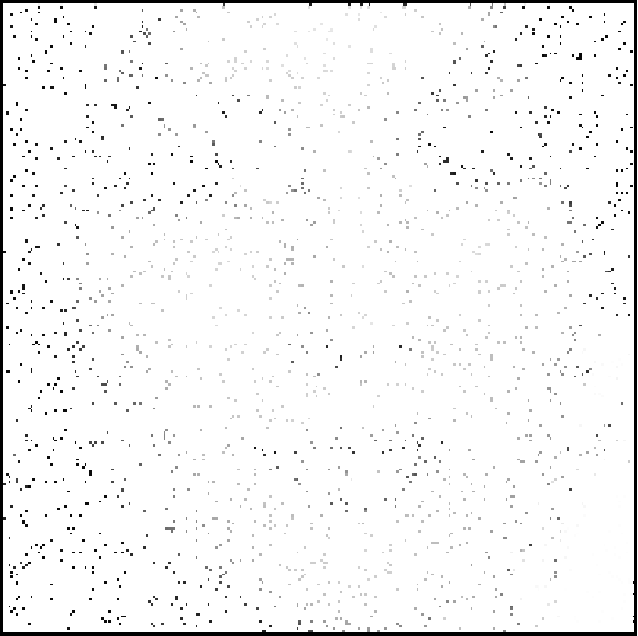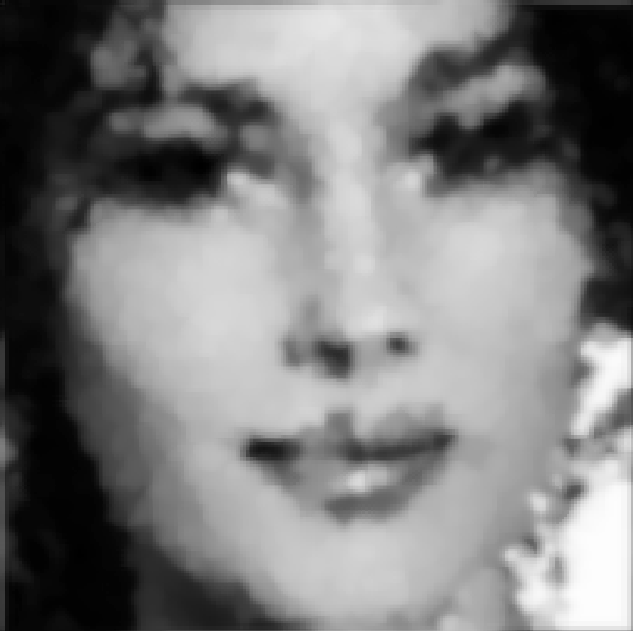# 2 Image reconstruction model

The geometric model for sound reconstruction was inspired by a sub-Riemannian model of the visual cortex V1 for image reconstruction. Therefore, a preliminary study of the image reconstruction was needed in order to understand the sound model and the challenges that are faced in adapting the initial model for sound reconstruction.

## 2.1 Neoro-geometric model of V1

My supervisors and their colleagues have presented a cortical-inspired image reconstruction algorithm [5,6] based on the Citti-Petitot-Sarti (CPS) model of the human primary visual cortex V1 [10,22].

The CPS model is based on the work of Hubel and Wiesel  who observed that there are groups of neurons sensitive to positions and directions. This has inspired Hoffman  to model V1 as a contact space (a three-dimensional manifold endowed with a smooth map). Since an image can be seen as a function $$f:\mathbb{R}^2\rightarrow\mathbb{R}_+$$ representing the grey level at given coordinates, the visual cortex adds the angle $$\theta\in P^1$$ of the tangent line to the curve, where $$P^1=\mathbb{R}/\pi\mathbb{Z}$$ is the projective line of non-directed angles. The model therefore lifts the image into $$\mathbb{R}^2\times P^1$$.

## 2.2 Wilson-Cowan model in V1

The Wilson-Cowan (WC) model  describes the evolution of neural activations, more precisely, it describes the evolution of excitatory and inhibitory activity in a synaptically coupled neuronal network.

The interaction between the hypercolumns in V1 can be described through the WC equations . An integro-differential equation describing the state $$a(x,\theta,t)$$ of a population of neurons with V1 coordinates $$x\in\mathbb{R}^2$$ and orientation $$\theta\in P^1$$ at time $$t>0$$ .

$\begin{equation} \frac{\partial}{\partial t}a(x,\theta,t) = -\alpha a(x,\theta,t) + \nu \int_{\mathbb{R}^2\times P^1} \omega(x,\theta\| x',\theta') \sigma(a(x',\theta',t)) \mathrm{d}x'\mathrm{d}\theta' + h(x,\theta,t) \end{equation}$Reconstruction of a 97% corrupted image (left: original, middle: corrupted, right: reconstructed)

### References


Marcelo Bertalmío, Luca Calatroni, Valentina Franceschi, Benedetta Franceschiello, and Dario Prandi. 2018. A cortical-inspired model for orientation-dependent contrast perception: A link with Wilson-Cowan equations. arXiv:1812.07425 [cs] (December 2018). Retrieved November 12, 2020 from http://arxiv.org/abs/1812.07425

Ugo Boscain, Roman Chertovskih, Jean-Paul Gauthier, Dario Prandi, and Alexey Remizov. 2017. Cortical-inspired image reconstruction via sub-Riemannian geometry and hypoelliptic diffusion. In SMAI 2017 - 8e Biennale Française des Mathématiques Appliquées et Industrielles, La Tremblade, France, 37–53. DOI:https://doi.org/10.1051/proc/201864037

Paul C. Bressloff and Jack D. Cowan. 2002. An Amplitude Equation Approach to Contextual Effects in Visual Cortex. Neural Computation 14, 3 (March 2002), 493–525. DOI:https://doi.org/10.1162/089976602317250870

G. Citti and A. Sarti. 2006. A Cortical Based Model of Perceptual Completion in the Roto-Translation Space. Journal of Mathematical Imaging and Vision 24, 3 (May 2006), 307–326. DOI:https://doi.org/10.1007/s10851-005-3630-2

William C. Hoffman. 1989. The visual cortex is a contact bundle. Applied Mathematics and Computation 32, 2 (August 1989), 137–167. DOI:https://doi.org/10.1016/0096-3003(89)90091-X

D. H. Hubel and T. N. Wiesel. 1959. Receptive fields of single neurones in the cat’s striate cortex. The Journal of Physiology 148, 3 (1959), 574–591. DOI:https://doi.org/10.1113/jphysiol.1959.sp006308

Jean Petitot and Yannick Tondut. 1999. Vers une neurogéométrie. Fibrations corticales, structures de contact et contours subjectifs modaux. Mathématiques et Sciences Humaines 145, (1999), 5–101. Retrieved August 13, 2021 from https://eudml.org/doc/94522

Hugh R. Wilson and Jack D. Cowan. 1972. Excitatory and Inhibitory Interactions in Localized Populations of Model Neurons. Biophysical Journal 12, 1 (January 1972), 1–24. DOI:https://doi.org/10.1016/S0006-3495(72)86068-5